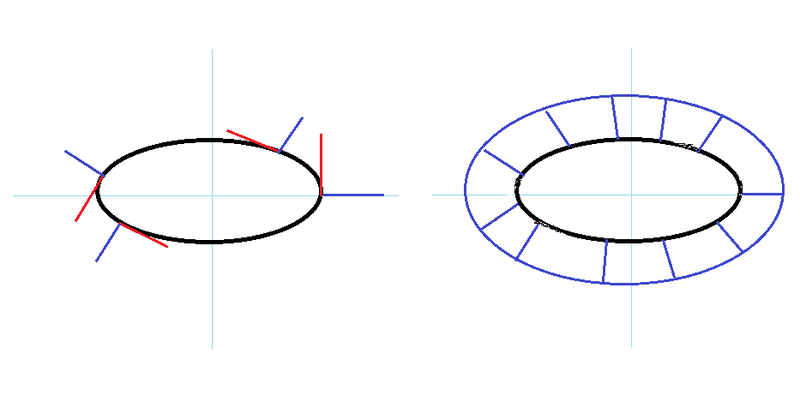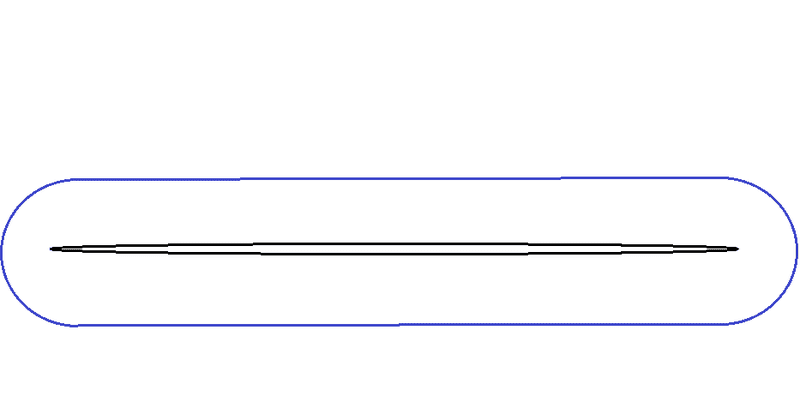# Equidistant curve of an ellipse

Draw an ellipse. Then draw a curve that is equidistant all the way around the outside of the ellipse. Is that new curve also an ellipse?

I've drawn it out and I can show examples where it's obviously not an ellipse, but I can't come up with a good non-visual explanation. I thought that maybe the new curve is also an ellipse that has the same foci as the smaller one, but that's also not the case.

I've attached a Geogebra file to show what I mean.

Can anyone help?

thanks.

#### Attachments

• EquidistantCurve.zip
4.8 KB · Views: 139

How do you define "equidistant"?

Here's the definition I assume you are using:

If C is a curve, and E is an ellipse, then C is "equidistant from E" if there is some positive real number r such that for all x in C, inf{|x-y| : y in E} = r.

This is actually an interesting question.

There is a minimum curve which is "inside" all other equidistant curves to E, and I assume this is what you are asking about. This is the curve C such that each point in C has a perpendicular distance of r from the ellipse E. In other words, each point x in C is distance r from some point y on E, and the tangent to y is perpendicular to the line between x and y. I think it's not to hard to show this curve is unique. Moreover, if any point is inside it, it must be closer than r to some point, so C is minimal, in some sense.

Now, let E be parametrized by the equation f(t) = (a*cost, b*sint).
Then f'(t) = (-a*sint, b*cost) -- this is the tangent vector at each point of E.

We are going about the ellipse E counterclockwise, so if we rotate this tangent vector by pi/2 clockwise, we will get a perpendicular vector to the tangent vector that points out of the ellipse.

We can simply use a rotation matrix: http://en.wikipedia.org/wiki/Rotation_matrix

In this case, theta will be -pi/2 (as we want to rotate clockwise, not counter clockwise). Our matrix will be:

[ cos(-pi/2) -sin(-pi/2)]
[ sin(-pi/2) cos(-pi/2)]

=

[0 1]
[-1 0]

When applied to our tangent vector, we get:
(b*cost,a*sint).

Of course, we need to scale it to be of length r. Let the function s be s(t) = r/sqrt((b*cost)^2 + (a*sint)^2). Note s is real-valued, not vector-valued.

Then our curve C is parametrized by:

g(t) = f(t) + (s(t)*b*cost, s(t)*a*sint)

Fully expanded, we have:

g(t) = [ a*cost + b*cost*r/sqrt((b*cost)^2 + (a*sint)^2), b*sint + a*sint*r/sqrt((b*cost)^2 + (a*sint)^2) ]

So, what you question boils down to is: does g(t) describe an ellipse?

Have fun!

OK, here are some diagrams:On the left is an ellipse centered about the origin. If we parametrize it, then for each point on the ellipse, we have a tangent vector (in red). This is just a non-zero vector which lies in the tangent line to the ellipse. In blue, we have constructed segments of length r perpendicular to the red vectors, pointing away from the inside of the ellipse.

On the right, the curve in blue is the resulting curve. This is the curve parametrized by g(t), and is "equidistant" of distance r from the ellipse.

It's actually now obvious that this curve is NOT an ellipse, unless the original ellipse happened to be a circle. Here's why:

Suppose you take a very wide, very thin ellipse, which is very close to just being a line. Then near the rightmost and leftmost points of the ellipse, a curve which is equidistant from the ellipse will look like a semicircle. However, near the middle of the curve, it's almost completely flat:Clearly this could not be an ellipse!

#### Attachments

lavinia
Gold Member
Draw an ellipse. Then draw a curve that is equidistant all the way around the outside of the ellipse. Is that new curve also an ellipse?

I've drawn it out and I can show examples where it's obviously not an ellipse, but I can't come up with a good non-visual explanation. I thought that maybe the new curve is also an ellipse that has the same foci as the smaller one, but that's also not the case.

I've attached a Geogebra file to show what I mean.

Can anyone help?

thanks.

i think this is right but ....

center the ellipse at the origin with its major and minor axes lying on the x and y coordinate axes. the four points along the major and minor axis that are equidistant to the axes along the normal direction uniquely determine an ellipse with the same allignment. but this ellipse is not equidistant along the normal direction as is easily seen from the trammel of archimedes.

see the wikipedia article

Hi, using the EquidistantCurve file from EebamXela I built an ellipse which has the same axes as the curve that is equidistant around the outside of the original ellipse to visualize the differences.

#### Attachments

• EquidistantCurvevsellipse.zip
8.7 KB · Views: 131